# Spectrum is sober

## Statement

### Topological statement

The spectrum of a commutative unital ring, with the usual topology, is a sober space: in other words, any irreducible closed subset is the closure of a one-point subset.

### Ring-theoretic statement

Any irreducible closed subset in the spectrum of a commutative unital ring corresponds to a prime ideal under the Galois correspondece between a ring and its spectrum.

## Proof

Given: A commutative unital ring$R$, its spectrum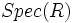$Spec(R)$, an irreducible closed subset$A$ of$Spec(R)$. Let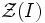$\mathcal{Z}(I)$, for an ideal$I$, denote the set of prime ideals containing$I$.

To prove: There is a prime ideal$P$ such that$A$ is precisely the set of all prime ideals containing$P$

Proof: By definition of closed set, there exists a unique radical ideal$P$ such that$A$ is precisely the set of prime ideals containing$P$. We need to show that$P$ is in fact prime. Suppose not. Then there exist ideals$J$ and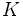$K$ of$R$ such that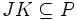$JK \subseteq P$ but neither$J$ nor$K$ is contained in$P$.

We now argue the following:

•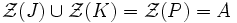$\mathcal{Z}(J) \cup \mathcal{Z}(K) = \mathcal{Z}(P) = A$: If$Q$ is a prime ideal containing$P$, then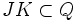$JK \subset Q$. But primeness of$Q$ forces$J \subset Q$ or$K \subset Q$, thus forcing$Q \in \mathcal{Z}(J) \cup \mathcal{Z}(K)$.
• Both are closed subsets: This is by definition
• Both are proper subsets: If$\mathcal{Z}(J) = \mathcal{Z}(P)$, then$P$ being a radical ideal, must be the radical of$J$. But that'd force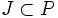$J \subset P$, a contradiction to assumption. Hence$\mathcal{Z}(J)$ must be a proper subset of$A$. A similar argument holds for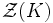$\mathcal{Z}(K)$.

Thus, we have expressed$A$ as a union of two proper closed subsets, a contradiction. Hence, our original assumption was false, and$P$ is prime.